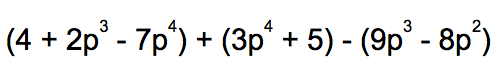Distributive Property and Factoring
Commutative and Associative
Multiplying Polynomials
Random
100

Distribute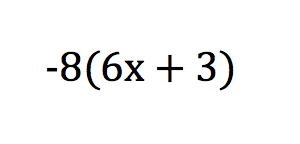-48x-24

100

Name the property and provide the actual expression answer.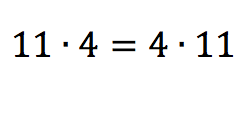commutative property of multiplication

100

add/subtract and combine like terms to simplify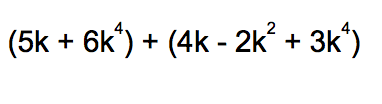9k4 - 2k2 + 9k

100

multiply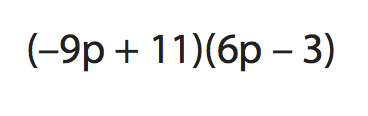–54p2 + 93p – 33

200

Distribute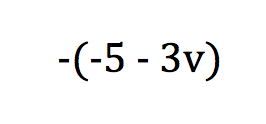3v+5

200

name the property that was used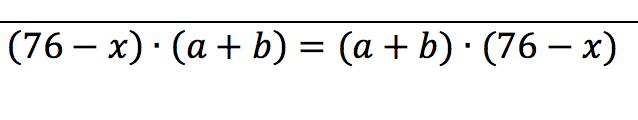commutative property of multiplication

200h5 - 3h3 + 13

200

multiply3p6q4 + 3p7 + 9q4 – 9p

300

Factor (find the GCF)9(x+4)

300

name the property that was used.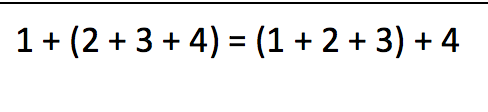3005p4 - 2p2 + 7p - 2

30018g8 + 18g6 + 34g4 + 16g2 + 2

400

Factor (find the GCF)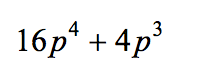4p3(4p+1)

400

name the property that was used.400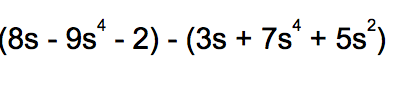- 16s4 - 5s2 + 5s - 2

400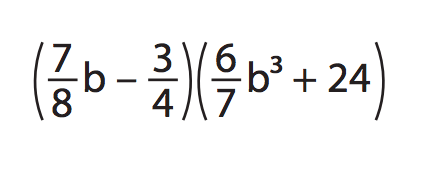3/4b- 9/14b+ 21b – 18

500

Factor-3k(k2-5k+2)

OR

3k(-k2+5k-2)

500

For the problem on the left, choose which answer A,B, or C represents an equivalent expression using the ASSOCIATIVE Property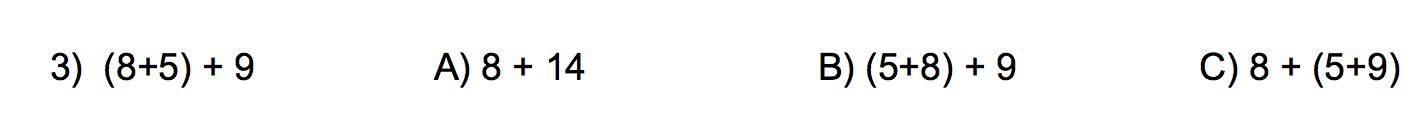C

500